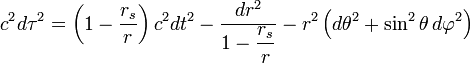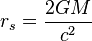# Time dilation (1 Viewer)

### Users Who Are Viewing This Thread (Users: 0, Guests: 1)

#### powerplayer

I was wondering how you would calculate the delta-T'(would it be correct to word delta-T' as change in time change?) for this example: (this is not a HW question)

observer A is at earth. observer B fly's 10 light years away at .5c to a non-rotating black hole 1 million times the mass of earth's star. B does a half orbit around the black hole at a distance of 1.1 times the swartschild radius. During the orbit B increase his velocity to stay in orbit and then returns home at .5c.

how much time has passed for B compared to A on the trip to the black hole?
how much time passed for B compared to A during the orbit?
how much total time passed for B compared to A?

I drew an illustration and put it as an attachment.

This is NOT a HW question. I simply made the example to give some life to the problem. if you don't show how to solve the problem, I wont care. I am really interested in how time dilation is effected by velocity and gravity at the same time. any and all contributions of knowledge or ideas on this subject are greatly appreciated . thanks in advance!!!

edit: Neglect earths gravity for any time dilation.

#### Attachments

• 15.2 KB Views: 122
Last edited:

#### powerplayer

Last edited by a moderator:

#### jambaugh

Gold Member
The way this problem would be solved is to write the Schwarzschild metric and integrate proper time along the space-time path.

Note your object must be under acceleration to keep it from speeding up as in falls into and to accelerate it out of the black hole's gravity well, and also to keep it in orbit that close.

You're going to likely need to integrate this numerically. Using the metric in a curved space-time setting will take into account all effects, velocity and gravitational so your reference is probably fine. I say all effects but there's only one "effect" Different paths through space-time have different proper time components. Some are in flat space-time and you say it's "a velocity effect" some are in curved space-time with zero relative velocities and you say it's "a gravitational effect" but all are "geometric effects".

[EDIT]
I assume from your drawing that you mean the object to "orbit" at 0.5c? As seen from Earth?

Last edited:

#### jambaugh

Gold Member
Working on some calculations:

I think it best to work in c=1 units, convert all distances to light-seconds (Ls).
The Schwarzschild radius of your BH is R=9.85 Ls. So the arc length of your half orbit path is 34.05 Ls.

$$d\tau^2 = \left(1 - R/r\right)dt^2 -\left(1-R/r)^{-1} dr^2 - r^2 d\theta^2$$

At r=1.1 R you get:
$$1-R/r = 0.090909,\quad \sqrt{1-R/r}=0.3015$$

For the half orbit at v=0.5 the observer time is
$$2\times 34.05 = 68.1 s$$
$$d\tau = \sqrt{1-R/r} dt - r d\theta$$
constancy allows simple integration:
$$\tau_{orb}= 0.3015 \times2\times 34.05 s - 34.05 s = -13.5s$$

This negative proper time means that your situation is unphysical. Due to the time dilation deep in the gravity well the object cannot travel at what appears to be 0.5c from the observer's (earth's) perspective.

Let's try it this way. Suppose the object travels at speed c.
Then dtau = 0 and 0.3015 dt = r dtheta. So to a distant observer light traveling 1.1R from your black hole is traveling at only 30.15% the speed of light.

In short your problem is ill posed. (Or I made an error. (Or GR is wrong.))

#### powerplayer

The way this problem would be solved is to write the Schwarzschild metric and integrate proper time along the space-time path.τ is the proper time (time measured by a clock moving with the particle) in seconds,
c is the speed of light in meters per second,
t is the time coordinate (measured by a stationary clock at infinity) in seconds,
r is the radial coordinate (circumference of a circle centered on the star divided by 2π) in meters,
θ is the colatitude (angle from North) in radians,
φ is the longitude in radians, and
rs is the Schwarzschild radius(^taken from Wikipedia of schwarzschild metric)

so I would integrate with respect to tau in the Schwarzschild metric for the orbit part of the journey?

would the straight paths be handled the same?

Note your object must be under acceleration to keep it from speeding up as in falls into and to accelerate it out of the black hole's gravity well, and also to keep it in orbit that close.
the wiki said something about the orbital velocity approaching c with that close of an orbit.
this is why a force is needed to maintain orbit?
also how would I calculate the force required to maintain my close orbit?
what direction would the force have to be maintain the orbit?

Using the metric in a curved space-time setting will take into account all effects, velocity and gravitational so your reference is probably fine.
so the Schwarzschild metric shows that time dilation due to velocity and gravity are related?
or if not then how are time dilation due to velocity and gravity related if at all?

also for the orbit part of the journey i was just proposing that the orbital velocity would be calculated from the mass of the black hole and the distance of the orbit, but it seems that is not the case in an extremes like this.

Thx

#### powerplayer

This negative proper time means that your situation is unphysical. Due to the time dilation deep in the gravity well the object cannot travel at what appears to be 0.5c from the observer's (earth's) perspective.
well the wiki did say that the orbit needs to be 3 times the schwarzschild radius? maybe this is why

#### jambaugh

Gold Member
If you are willing to allow the closest distance from the black hole to be 2 Schwarzschild radii then the object will be traveling at c but appear to be traveling at 1/2 c so you can figure the time difference for that leg to be the full distance=time of flight or 61.9 seconds.

The other two legs will be hairy to integrate if you want exact tangential flights. However going in and then out radially should give a good estimate.

At v = 1/2 you get dt = 2dr.

$$d\tau =R \sqrt{ 2(1-R/r) - (1-R/r)^{-1}} dr$$

Integrate from 31557600+2R to 2R and double to get proper time of flight. It would be best here to work in multiples of the Sch.Rad R. 1 lightyear = 3203037 R so integrate from 2 to 3203039 the following:

$$\tau_{leg} = R\int_{2}^{3203037}dx\cdot\sqrt{2(1-1/x) - (1-1/x)^{-1}}$$

Compare that with the 2 years (2 times 31557600 sec) the observe watches the object travel 1 light-year at apparent 1/2 c.

 oops forgot to square the 2 inside the radical! Should read:
$$d\tau = \sqrt{ 4(1-R/r) - (1-R/r)^{-1}} dr$$

$$\tau_{leg} = R\int_{2}^{3203037}dx\cdot\sqrt{4(1-1/x) - (1-1/x)^{-1}}$$
[edit again]
Forgot to put R in dimensionless integrals (note that's R in light-seconds = 9.8524s)

Last edited:

#### jambaugh

Gold Member
well the wiki did say that the orbit needs to be 3 times the schwarzschild radius? maybe this is why
For a free orbit it needs to be 3R out for stability. It will otherwise spiral inward. As you posed the problem I must assume the object is being artificially accelerated by some external force to keep it along your paths at your speeds. So this 3R requirement doesn't apply.

Rather what occurs is that a distance observer watching an object that near a black hole will see an extreme time dilation. They see, for example, light traveling at 30% c as they measure time and distance.

#### jambaugh

Gold Member
Final remark. I used a crude numerical technique and got about 8.9 seconds more time lost on each of the inward and outward legs due to the BH's effect at 2R.

This is small relative to the 1.4641 years less the object experiences for the two legs (4 year trip) dtau / dt = sqrt(3)/2.

#### jambaugh

Gold Member
Final Final remark...
An interesting aspect of this problem is that to maintain the 0.5c apparent speed the object will have to be externally accelerated away from the BH for most of the inward leg to keep it from speeding up due to gravity but towards the end it must be accelerated toward the BH to overcome the increasing gravitational time-dilation in order to appear to be traveling a constant 0.5c from the distant observer's point of view.

Now that's an interesting calculation. What is the acceleration the object experiences so as to keep it at this apparent 0.5 c velocity?

### The Physics Forums Way

We Value Quality
• Topics based on mainstream science
• Proper English grammar and spelling
We Value Civility
• Positive and compassionate attitudes
• Patience while debating
We Value Productivity
• Disciplined to remain on-topic
• Recognition of own weaknesses
• Solo and co-op problem solving